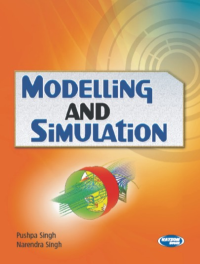•Modelling And Simulation

In Stock
ISBN 978-93-80027-22-7 Pushpa Singh, Narendra Singh S.K. Kataria & Sons 2008
Rs 250.00 Rs 225.00

CONTENTS

• Introduction to System and Models • System Study • System Simulation • Input Data Model • Process and Validation of Modeling and Simulation • Basic of Probability • Continuous and Discrete System Simulation • System Dynamics • Random Number Generation • Simulation of Queuing System • Arrival Patterns and Discrete System Simulation • Simulation Language • GPSS • Estimation and Output Analysis of Simulation • Simulation of PERT Network • Real World Application of Simulation • Appendices • Model Test Papers • Examination Papers • Index.

• Author : Pushpa Singh, Narendra Singh
• Publisher : S.K. Kataria & Sons
• Publishing Year : 2008